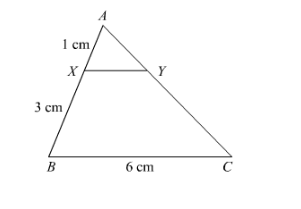# In the given figure, XY || BC. Find the length of XY.

Question:

In the given figure, XY || BC. Find the length of XY.Solution:

It is given that $X Y \| B C$.

$A X=1 \mathrm{~cm}, X B=3 \mathrm{~cm}$ and $B C=6 \mathrm{~cm}$

We have to find $X Y$.

Since $\triangle A X Y \sim \triangle A B C$

$\Rightarrow \frac{X Y}{B C}=\frac{A X}{A B}(A B=A X+X B=4)$

So

$\frac{X Y}{6 \mathrm{~cm}}=\frac{1 \mathrm{~cm}}{4 \mathrm{~cm}}$

$X Y=\frac{6 \mathrm{~cm}}{4 \mathrm{~cm}}$

$=1.5 \mathrm{~cm}$

Hence, $X Y=1.5 \mathrm{~cm}$